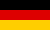Bri's worldelectronics, programming and more

deutschControl theory: control loop optimization with iteration

For a stable control loop the control parameters have to be set that no oscillations will occur. There are several methods to estimate the parameters for a stable control loop from oscillation period or control path step response.

With support of computer it is possible to use iterative methods to adapt the step response of the control loop to the desired curve. For this purpose a weighting function that depends on the control parameters is used to measure the deviation from the ideal curve. An iterative search algorithm is used to find the minimum of this weighting function. The greatest difficulty is that the weighting function does not have only one minimum but many local minima.

As iteration methods only those are applicable that do not use derivatives because the weighting function is not derivable in generally. I use the local variation and differential evolution as iteration methods for this application.

Differential evolution

The differential evolution is a variant of the genetic algorithms, which is optimized especially for functions with real parameters. It is an intelligent random-based search method.

• search covers the whole parameter space
• may find the global minimum
• does not find only a local minimum
• high calculation effort
• does not find the exact minimum because it is random-based

Local variation

This iterative search method alters the function parameters to find step by step better values for the minimum.

• less calculation effort
• finds the local minimum exactly
• finds only local minima
• needs a start value

Control loop

First you have to define the control loop. This is done by selecting the control element types in the table below. The control elements time and gain parameters can be changed in the columns next to the control element type. The Start button is used to draw the control loop step response.

Control path parameters

Number Control element Parameter K Parameter T Parameter D Transfer function
1
2
3
4

Step response simulation parameters

The step response is used to optimize the control loop controller. The weighting function measures how good the step response fits the desired ideal curve.

 Step height Step time Simulation end time

PID controller parameters

This PID controller parameters are used as start values for the local variation method. The differential evolution does not need start values. Therefore it is a good idea to use the differential evolution first to estimate the parameters. The local variation can be used afterwards to improve the result.

Controller Parameter Kp Parameter Ki Parameter Kd Limit u min Limit u max Transfer function
PID $$K_p + \frac{K_i}{s} +K_d \cdot s$$

Parameters for the PID controller optimization

The weighting function used for optimization consists of three parts. The first part is the deviation of the output y(t) from the control loop input w(t) as the sum of the error squares. The weighting for this part is 1. The other parts are the output overshot and the output value of the controller.

 Maximum number iteration steps Maximum overshot in percent Weighting controller output Weighting overshot